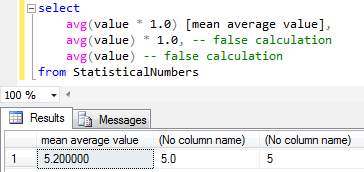SQL Server and T-SQL Development Tutorials Development resources, articles, tutorials, code samples and tools and downloads for ASP.Net, SQL Server, R Script, Windows, Windows Phone, AWS, SAP HANA and ABAP, like SAP UI5, Screen Personas, etc.
 Home Articles News IT Jobs Tools Sample Chapters Trainers Blogs Forums Photos Files

SQL Server 2019 Installation

# Calculate Mean Value in SQL Server

SQL programmers can calculate mean value of a numeric array in SQL Server by using SQL Server AVG() aggregate function. Transact-SQL developers or database administrators can use mean value calculation method on any numeric table column in a SQL Server database as shown in this SQL tutorial.

First of all let SQL database administrators create database table to store sample numeric values where programmers can calculate the mean value of the numeric values.

``` create table StatisticalNumbers(  value int ) ```

Let's now generate sample data and populate sql table.
SQL developers and database administrators can to calculate the mean value of the values stored in this table column. Here is a SQL script which will create 5 random numbers using SQL Rand() function and insert into database table.

``` declare @i int = 1 while @i <= 5 -- count of sample numbers begin  insert into StatisticalNumbers SELECT RAND()*10 -- (10-1) is max number  set @i=@i+1 end ```

Here is the source codes of the SQL Select script which can be used to calculate the mean value of a numeric value list given on SQL Server.
What is important about the below SQL mean value calculation using AVG() SQL aggregate function is multiplying the values with 1.0 beforehand for decimal value result. As seen below the following two average calculations return integer values losing the decimal points return false values.

``` select  avg(value * 1.0) [mean average value],  avg(value) * 1.0, -- false calculation  avg(value) -- false calculation from StatisticalNumbers ```

Here is a screenshot of the above mean calculation or average calculation in SQL Server.Calculate mean value of an array in SQL Server

It is also possible to create a user defined SQL function which takes the numeric values as a string input parameter formed of concatenated numeric values and returns the mean value as follows.

Since SQL aggregate function does not accept multiple values I had to create this SQL mean value calculation function.

In the Mean calculation function, a second user defined SQL function dbo.split() is used to split concatenated numeric values which are passed as input to the SQL mean function. SQL Server developers and administrators can check SQL tutorial SQL Split function for the source codes of the helper function.

``` create function udf_calculate_mean (  @numericValueList varchar(max) ) returns float as begin  declare @mean float  select @mean = avg(cast(val as int) * 1.0 ) from dbo.Split(@numericValueList,',')  return @mean end ```

And this sample SQL function can be used to calculate mean value of a numeric list or average value of items as given in the following example on SQL Server

``` declare @numericValueList varchar(max) = '1,4,0,2' select dbo.udf_calculate_mean(@numericValueList) [Mean Value] ```Mean value (average) calculation function for SQL Server developers

Related SQL Resources

SQL Server Articles

SQL Server 2012

SQL Server Tools

SQL Blog

MS SQL Server Forums